# Rectangle

A rectangle is a four-sided flat shape where every angle is a right angle (90°).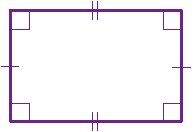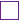means "right angle"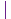are equal sidesare equal sides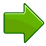Each internal angle is 90°Opposite sides are parallel and of equal length (so it is a Parallelogram).

Play with a rectangle:

## Area of a RectangleThe Area is the width times the height: Area = w × h

Area= 6 m × 3 m
= 18 m2

## Perimeter of a Rectangle

The Perimeter is the distance around the edges.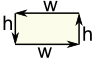The Perimeter is 2 times the (width + height): Perimeter = 2(w+h)

### Example: A rectangle has a width of 12 cm, and a height of 5 cm, what is its Perimeter?

Perimeter = 2 × (12 cm + 5 cm)
= 2 × 17 cm
= 34 cm

## Diagonals of a Rectangle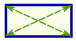A rectangle has two diagonals, they are equal in length and intersect in the middle.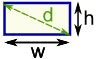The Diagonal is the square root of (width squared + height squared): Diagonal "d" = √(w2 + h2)

### Example: A rectangle has a width of 12 cm, and a height of 5 cm, what is the length of a diagonal?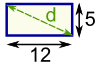d = √(52 + 122)
= √(25 + 144)
= √169
= 13 cm## Golden Rectangle

There is also a special rectangle called the Golden Rectangle: